• FunctionGraphDrawing 绘制函数图的程序，包括一元函数和二元函数 在这里查看更多： 一些截图 一元函数图 二元函数图 解析函数字符串C#
• 遗传算法优化一元函数。遗传算法优化一元函数遗传算法优化一元函数遗传算法优化
• 简单一元函数优化， Sheffield的遗传算法工具箱，数学建模算法大全MATLAB智能算法
• 下面是我考研复习数学的时候做的微积分知识点思维导图，也和大家分享一下，内容包括极限与连续，一元函数微分学，一元函数积分学，多元函数微分学以及微分方程 极限与连续 一元函数微分学 一元函数积分学 多元...
最近学最优化，涉及许多高数的知识，顺便复习一下。
下面是我考研复习数学的时候做的微积分知识点思维导图，也和大家分享一下，内容包括极限与连续，一元函数微分学，一元函数积分学，多元函数微分学以及微分方程
极限与连续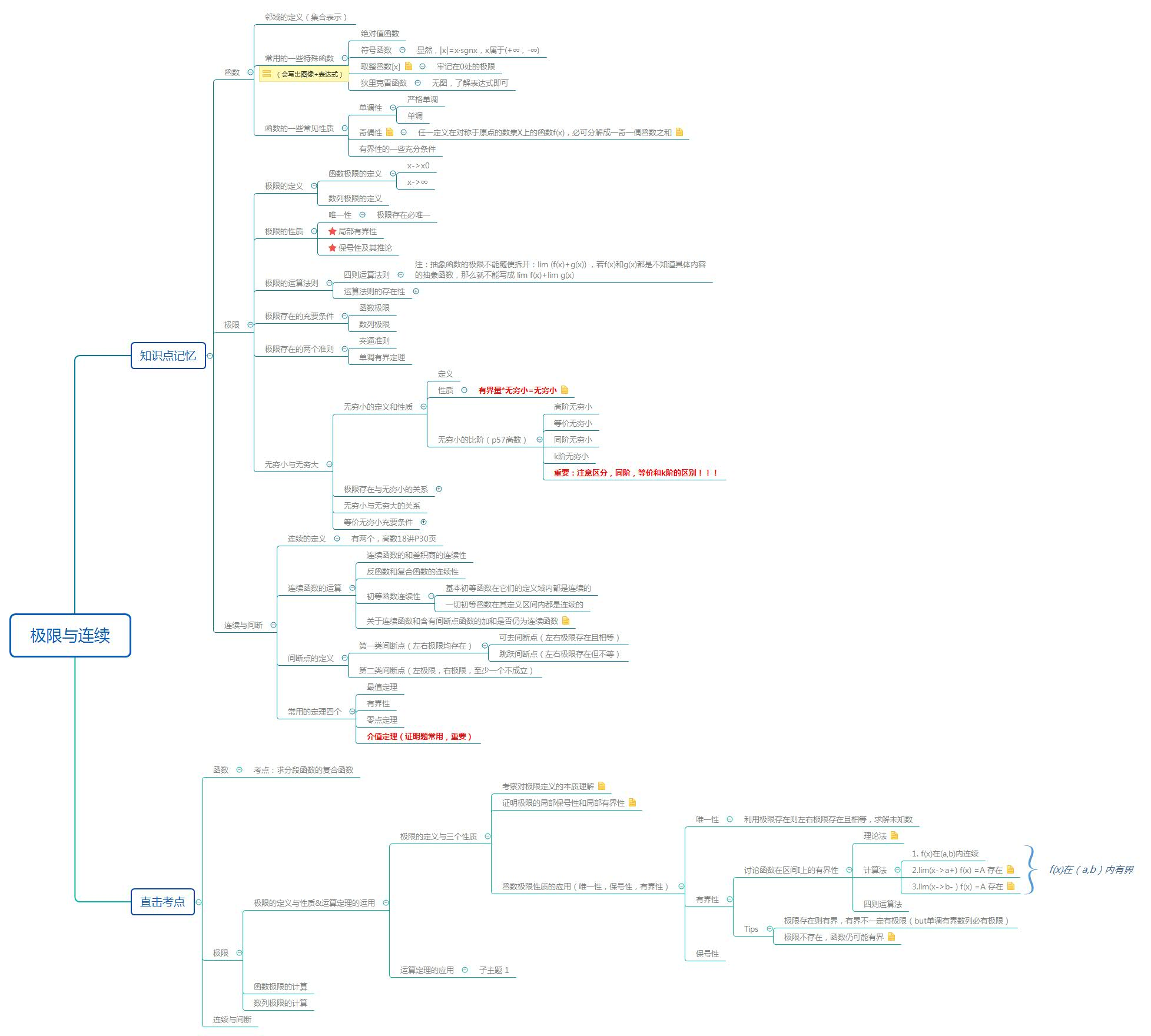一元函数微分学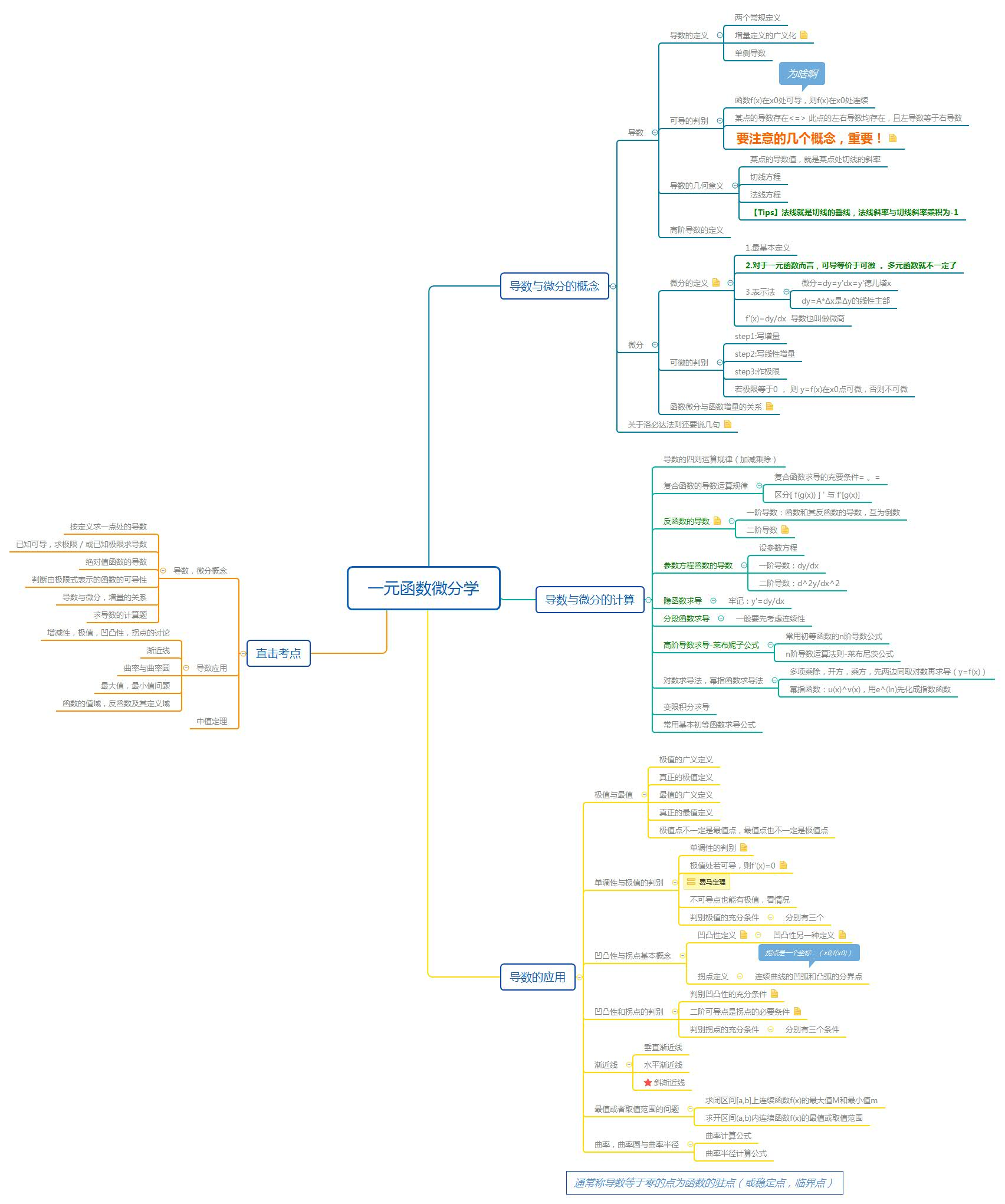一元函数积分学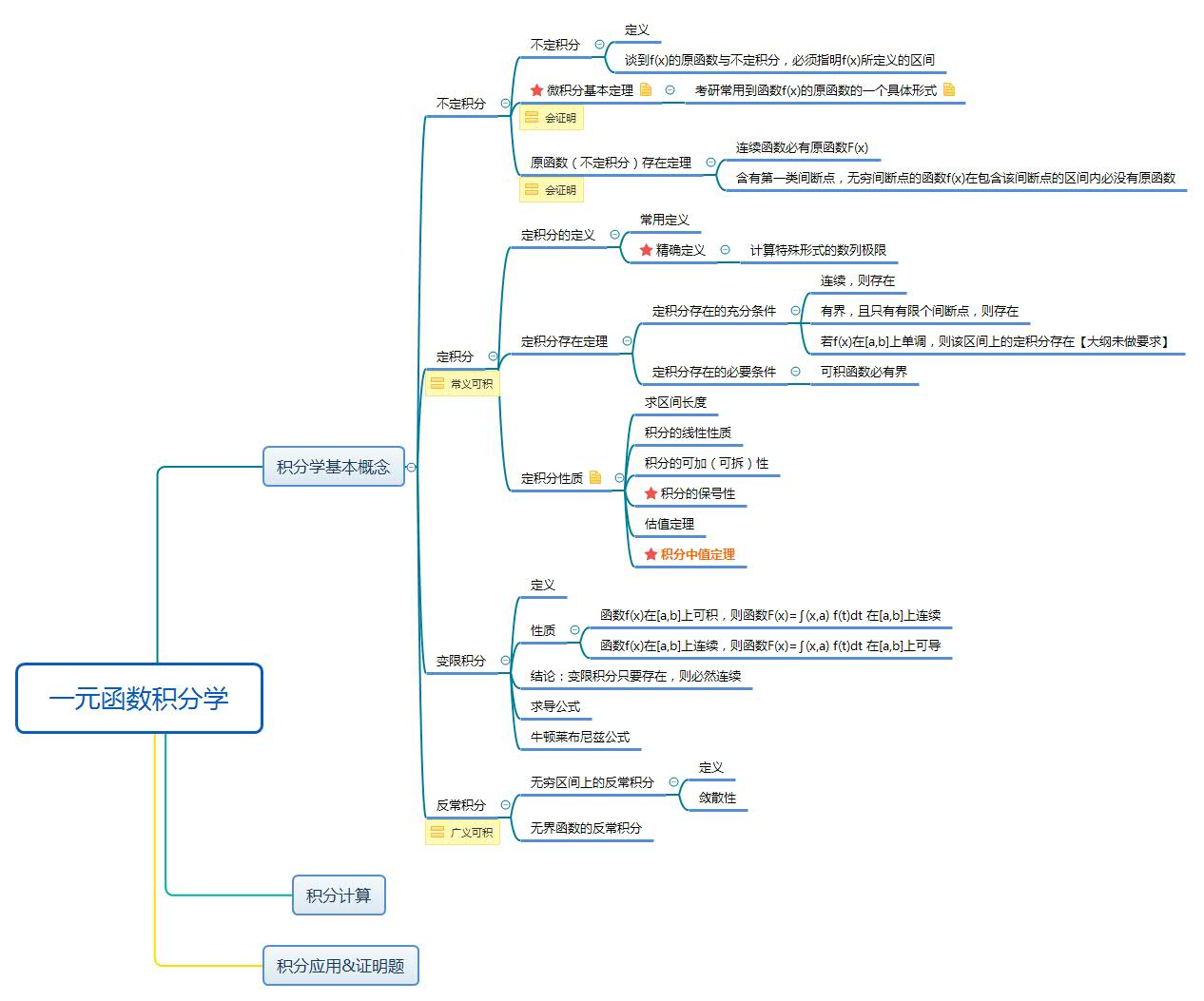多元函数微分学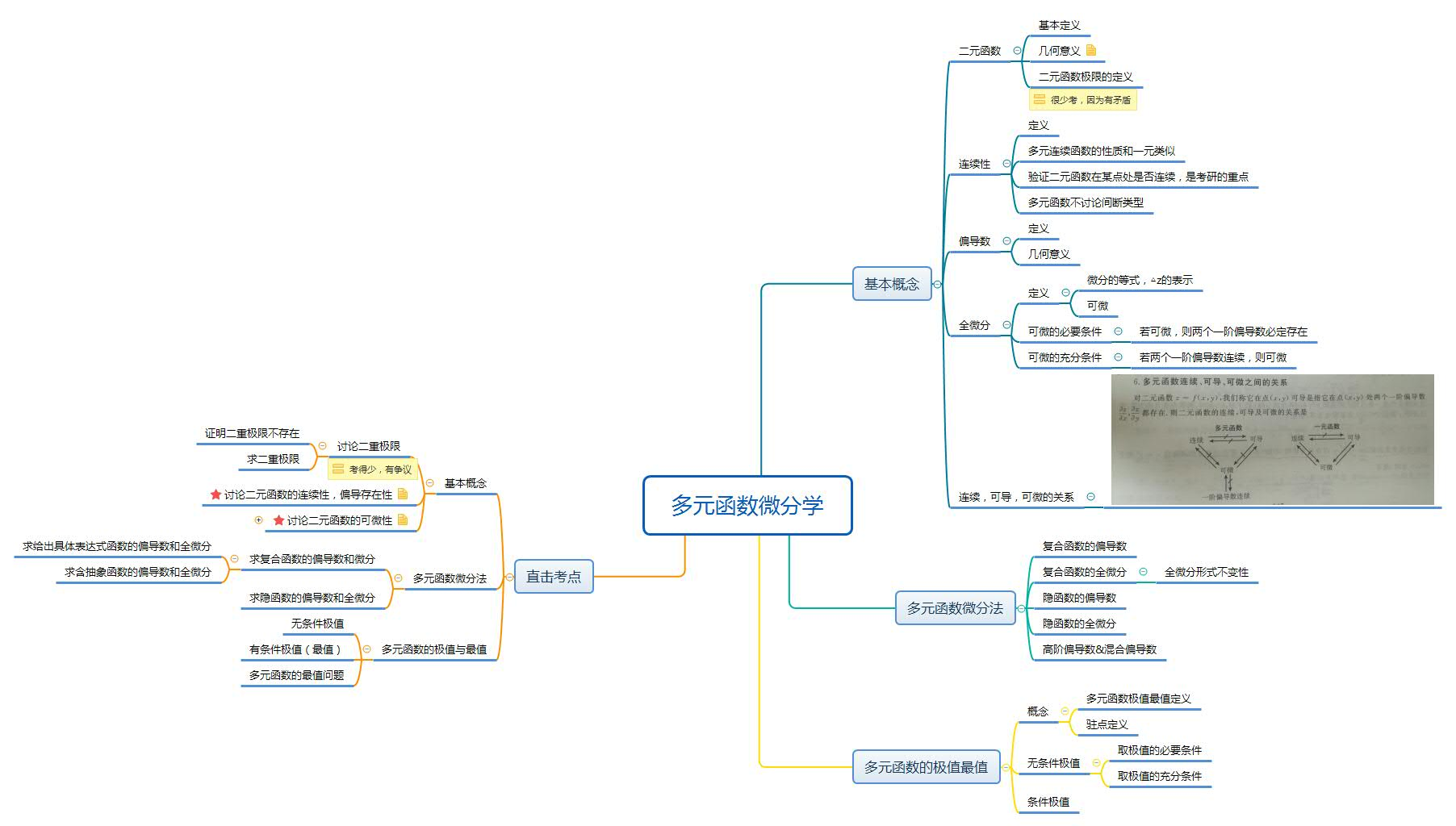微分方程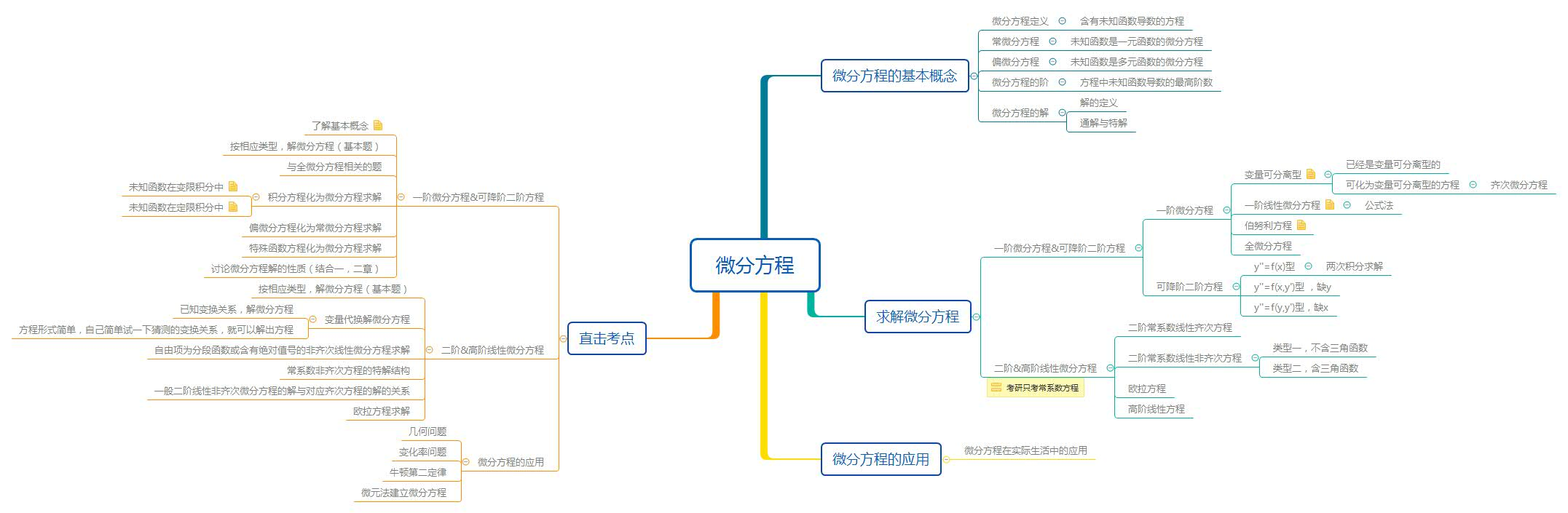展开全文• #include <iostream> #include <vector> #include <algorithm>...STL中的一元函数基类 template<class _A, class _R> struct unary_function { typedef _A argument_ty...
#include <iostream>
#include <vector>
#include <algorithm>

using namespace std;

/*
STL中的一元函数基类
template<class _A, class _R>
struct unary_function
{
typedef _A argument_type;
typedef _R result_type;
};
*/
//应用STL模板一元函数必须从unary_function基类派生

template<class _In, class _Out>
class CSum : public unary_function<_In, _Out>
{
public:
CSum()
{
m_sum = 0;
}

CSum(_Out sum)
{
m_sum = sum;
}

void operator()(_In n)
{
m_sum += n;
}

_Out GetSum()const
{
return m_sum;
}

private:
_Out m_sum;
};

int main()
{
vector<int> vi;
for(int i = 1; i <= 100; i++)
{
vi.push_back(i);
}
CSum<int, int> cs = for_each(vi.begin(), vi.end(), CSum<int, int>());	//无参
CSum<int, int> cs_1 = for_each(vi.begin(), vi.end(), CSum<int, int>(100));  //有参
cout << cs.GetSum() << endl;
cout << cs_1.GetSum() << endl;

vector<float> vif;
float f = 1.3f;
for(int i = 1; i <= 100; i++)
{
vif.push_back(f);
f += 1.0f;
}
CSum<float, float> cs0 = for_each(vi.begin(), vi.end(), CSum<float, float>());
cout << cs0.GetSum() << endl;

return 0;
}




展开全文stl
• 主要是有关一元函数的极值求法和最值求解讲解，希望可以帮助到大家！
• 一元函数对象：函数参数1个 二元函数对象：函数参数2个； 一元谓词对象：函数参数1个，函数返回值是bool类型，可以作为一个判断式，谓词可以是一个仿函数，也可以是一个回调函数； 二元谓词对象：函数参数2个，函数...
一元函数对象：函数参数1个；
二元函数对象：函数参数2个；
一元谓词函数对象：函数参数1个，函数返回值是bool类型；
二元谓词函数对象：函数参数2个，函数返回值是bool类型；
之所以给返回布尔类型的函数对象专门命名，是因为谓词函数对象是用来为算法判断来服务的；
事实上，无论是普通函数指针、函数对象、lambda表达式，都是为STL中的算法服务的。
例1：顺便分析了STL中accumulate算法的源码
#include<iostream>
#include<functional>
#include<numeric>
using namespace std;

// TEMPLATE STRUCT plus
template<class _Ty = void>
struct my_plus
{	// functor for operator+
typedef _Ty first_argument_type;
typedef _Ty second_argument_type;
typedef _Ty result_type;

constexpr _Ty operator()(const _Ty& _Left, const _Ty& _Right) const
{	// apply operator+ to operands
return (_Left + _Right);
}
};

template<class _InIt,
class _Ty,
class _Fn2> inline
_Ty my_accumulate(_InIt _First, _InIt _Last, _Ty _Val, _Fn2 _Func)
{	// return sum of _Val and all in [_First, _Last), using _Func
for (; _First != _Last; ++_First)
_Val = _Func(_Val, *_First);
return (_Val);
}

// FUNCTION TEMPLATE accumulate
template<class _InIt,
class _Ty> inline
_Ty my_accumulate(_InIt _First, _InIt _Last, _Ty _Val)
{	// return sum of _Val and all in [_First, _Last)
return (my_accumulate(_First, _Last, _Val, my_plus<_Ty>()));
}
int main() {
//二元函数对象的使用
int a[] = { 1,2,3,4,5 };
const int N = sizeof(a) / sizeof(int);
cout << my_accumulate(a, a + N, 0) << endl;//15
cout << my_accumulate(a, a + N, 1, multiplies<int>()) << endl;//120

//一元函数对象的使用
//auto fp1 = negate<int>();
negate<int> fp1 = negate<int>();
cout << fp1(10) << endl;//-10

//二元谓词对象的使用
cout << equal_to<double>()(6.6, 6.6) << endl;//1
cout << greater<double>()(6.6, 6.5) << endl;//1

system("pause");
return 0;
}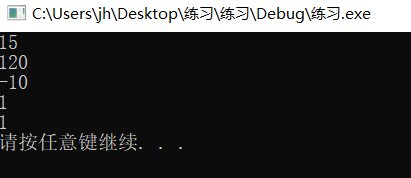例2：一元谓词函数对象的使用
#include<iostream>
#include<functional>
#include<numeric>
#include<algorithm>
using namespace std;
template <typename T>
class IsDiv
{
public:
IsDiv(const T divisor)
{
this->divisor = divisor;
}

bool operator()(T &t)
{
return (t%divisor) == 0;
}
private:
T divisor;
};

int main()
{
vector<int> v1;
for (int i = 11; i < 20; i++)
{
v1.push_back(i);
}
int divisor = 5;

vector<int>::iterator it = find_if(v1.begin(), v1.end(), IsDiv<int>(divisor));
if (it == v1.end())
{
cout << "不能被5整除" << endl;
}
else
{
cout << "第一个能被" << divisor << "整除的数：" << *it << endl;
}
system("pause");
return 0;

}例3：
#include<iostream>
#include<functional>
#include<numeric>
#include<algorithm>
#include<vector>
using namespace std;

template<class T>
bool MyCompare(const T &a, const T &b)
{
return a < b;
}

template<class T>
void MyPrint1(const T&i)
{
cout << i << endl;
}
template<class T>
class MyPrint2 {
public:
void operator()(const T&i)
{
cout << i << endl;
}
};

int main()
{
vector<int> v1;
for (int i = 0; i < 10; i++)
{
v1.push_back(rand() % 100);
}
for_each(v1.begin(), v1.end(), MyPrint1<int>);

cout << "After sorted：" << endl;
sort(v1.begin(), v1.end(), MyCompare<int>);
for_each(v1.begin(), v1.end(), MyPrint2<int>());

system("pause");
return 0;
}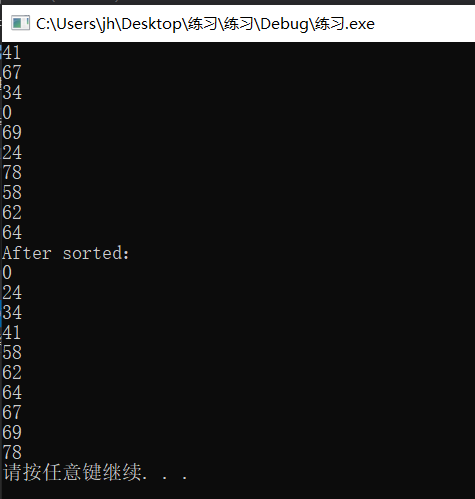例4：lambda表达式是一种可调用对象。C++中可调用对象不仅有函数指针、重载了"()"运算符的类即functor、lambda表达式。
#include<iostream>
#include<vector>
#include<algorithm>
using namespace std;
int main()
{
vector<int>vi = { 5,28,50,83,70,590,245,59,24 };
int x = 30, y = 100;
for (int&elem : vi)
{
cout << elem << " ";
}
cout << endl;
//remove_if()将所有应该移除的元素都移动到了容器尾部并返回一个分界的迭代器
vector<int>::iterator ret_it = remove_if(vi.begin(), vi.end(), [x, y](const int& n) ->bool {return x < n&&n < y; });

for (int&elem : vi)
{
cout << elem << " ";
}
cout << endl;

vi.erase(ret_it, vi.end());
//或者直接合在一起写成下面的句子：
//vi.erase(remove_if(vi.begin(), vi.end(), [x, y](int n) {return x < n&&n < y; }), vi.end());

for (int&elem : vi)
{
cout << elem << " ";
}
cout << endl;
system("pause");

return 0;
}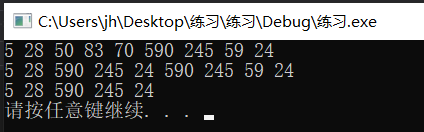例5：函数适配器binder2nd的源码分析
自己已经把注释写的很清楚了，很好懂。
其实本质就是调用bind2nd函数（创建）返回一个my_binder2nd类的对象，my_binder2nd是仿函数适配器functor adapter。
#include<iostream>
#include<vector>
#include<functional>
#include<algorithm>
using namespace std;
// TEMPLATE STRUCT less
template<class _Ty = void>
struct my_less
{	// functor for operator<
typedef _Ty first_argument_type;
typedef _Ty second_argument_type;
typedef bool result_type;

constexpr bool operator()(const _Ty& _Left, const _Ty& _Right) const
{	// apply operator< to operands
return (_Left < _Right);
}
};

template<class Operation>
class my_binder2nd//仿函数适配器functor adapter
:public unary_function<typename Operation::first_argument_type, typename Operation::second_argument_type>
{
protected://两个数据成员
Operation op;//二元函数对象
typename Operation::second_argument_type value;
public:
//constructor
my_binder2nd(const Operation &obj, const typename Operation::second_argument_type &val) :op(obj), value(val) {}
typename Operation::result_type//函数返回类型
operator()(typename Operation::first_argument_type&x)const
{
return op(x, value);
}
};

template<class Operation, class T>
inline my_binder2nd<Operation>my_bind2nd(const Operation&op, const T&x)//仿函数适配器辅助函数（自己写一个my_bind2nd函数模板，目的是为了创建一个my_binder2nd的对象），主要是借助函数模板具有可以自动推导形参类型的功能
{
typedef typename Operation::second_argument_type agr2_type;
return my_binder2nd<Operation>(op, agr2_type(x));//调用my_binder2nd的constructor创建my_binder2nd的对象
}
int main()
{
vector<int>v = { 5,10,20,30,40,50 };
cout << count_if(v.begin(), v.end(), my_bind2nd(my_less<int>(), 40)) << endl;
//cout << count_if(v.begin(), v.end(), my_bind2nd(my_less<int>(), 40.1)) << endl;
system("pause");
return 0;
}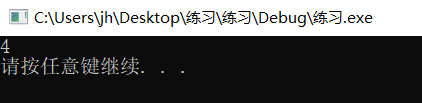展开全文• 一元函数微分学 主要涉及两方面: 概念类。主要是定义，通过一个式子来判断它的导数(存不存在、是多少)。 计算类。(包括很多 分段函数 复合函数 反函数 参数方程 隐函数 对数求导 幂指函数 高阶导数 变限积分求导) ...
一元函数微分学
主要涉及两方面:

概念类。主要是定义，通过一个式子来判断它的导数(存不存在、是多少)。
计算类。(包括很多  分段函数  复合函数  反函数  参数方程  隐函数  对数求导  幂指函数  高阶导数  变限积分求导)  首先要掌握基本求导公式。

导数 概念类：

导数的定义：两种式子。一个是△x→0，一个是x→x0。    需要注意的是当用一个复杂的式子来替换△x的情况!!
导数与极限的关系：一一对应。左导数代表左极限，右导数代表右极限。
导数的几何意义：切线斜率。
可导的充分必要条件是左右导数均存在且相等。   导数等于∞不算存在!!
高阶导数的概念记住。

微分的概念：（△x=dx）

△y＝f(x0+△x)-f(x0)     这个叫增量。
dy=A△x＝f’(x0)△x     这个叫线性增量。
判断可微的方法，因为可微与可导是互为充要条件，所以可以将判断可微转化为判断可导。

重头戏是关于导数、微分的计算：

四则运算（非常简单  记住就好）
分段函数的导数：分段点处使用定义，一定要使用定义做!!!（判断左右导数是否相等），非分段点可以直接使用求导公式。
复合函数的导数与微分形式的不变性。
反函数的导数→反函数的二阶导（有公式  背住）
参数函数的导数→参数函数的二阶导（简单）
隐函数的导数→隐函数的二阶导（记住不要忘记y对x的求导）
对数求导法：适用于非常多项相乘除，原本的式子非常不好做。用对数将乘除化成加减，将指数化成系数。
幂指函数求导法：先用公式化成e为底的指数函数。
高阶导数：有公式，背上，会推广。
还有泰勒公式，要记住任何一个无穷阶可导的函数都可以写成无限求和的形式。


展开全文• [xmin, ymin] = fminbnd(fun, x1, x2) 用于求解一元函数 fun 的极小值 其中，fun是目标函数，[x1，x2]是搜索区间，xmin、ymin分别是目标函数的极小点、极小值
• 本资源为.zip压缩包，...注：源文件中的一元函数需要你自己修改，修改方式为在target.m文件中重新定义你自己的一元函数，且需要在gene.m文件中的boundsbegin变量、boundsend变量重新赋值你所定义的一元函数的上下界。
• 本文实例为大家分享了C++实现二分法求连续一元函数根的具体代码，供大家参考，具体内容如下 设计一个用二分法求连续一元函数根的通用函数solve 此函数有三个参数： 第一个是函数指针，指向所要求根的连续函数 第二...
• 一元函数对象：函数参数1个； 二元函数对象：函数参数2个； 一元谓词 函数参数1个，函数返回值是bool类型，可以作为一个判断式 谓词可以使一个仿函数，也可以是一个回调函数。 二元谓词 函数参数2个，函数返回值是...
• 一元函数对象：函数参数1个 二元函数对象：函数参数2个 一元谓词 函数参数1个，函数返回值是bool类型，可以作为一个判断式，谓词可以是一个仿函数，也可以是一个回调函数。 二元谓词 函数参数2个，函数返回值是...二元谓词
• 这是一部关于matlab的教学资料是关于一元函数微积分学的知识matlab
• 南开大学AMTD0013一元函数微分（信）课程2014-2016考试真题，内含真题试卷以及解答，仅供参考使用微积分
• 一元函数、多元函数的泰勒公式 转载于:https://www.cnblogs.com/liuys635/p/11181330.html
• 应用matlab建立粒子群算法来解决复杂一元函数求解问题。
• 通过最简单的一元函数的求解来详细解释如何通过粒子群算法进行编程，注释详细。
• 一元函数在区间上连续的一个充要条件.pdfv一元函数在区间上连续的一个充要条件.pdf一元函数在区间上连续的一个充要条件.pdf一元函数在区间上连续的一个充要条件.pdf
• 牛顿法求解一元函数 对于一个简单的一元方程我们可以通过代数运算来求解，但是对于一个非线性的复杂一元函数例如−2x5−8x2+sin(x∗x)−2x=0−2x5−8x2+sin(x∗x)−2x=0-2x^5 - 8x^2 + sin(x*x) - 2^x=0这样的方程...表达式求值
• Python遗传算法求一元函数最大值前言代码后记参考文献 前言 最近接触遗传算法，参考了众多例子，有些又不尽然对，所以自己边理解边修改，然后写出了下面这堆传说中的屎山。。。 PS1：遗传算法原理啥的太多了，就不...
• matlab源码求一元函数 matlab 强制关闭当前程序(万能保险) Ctrl+c 常见函数 format 三角函数 直接sin和cos是对应弧度制 sind和cosd才是对应角度 exp函数是e^x abs求绝对值 取整函数 round 四舍五入 ceil 向上取整 ......